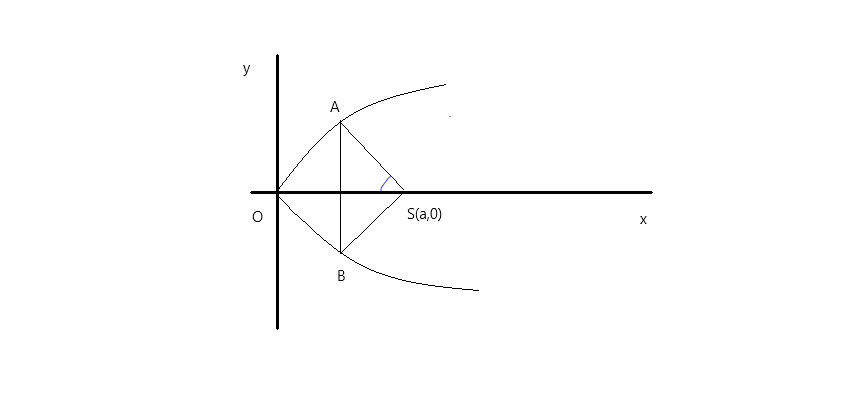Courses
Courses for Kids
Free study material
Free LIVE classes
MoreLIVE
Join Vedantu’s FREE Mastercalss

# An equilateral triangle SAB is inscribed in the parabola ${y^2} = 4ax$ having its focus at S. If chord AB lies towards the left of S, then side length of the triangle isA. $2a\left( {2 - \sqrt 3 } \right)$B. $4a\left( {2 - \sqrt 3 } \right)$C. $a\left( {2 - \sqrt 3 } \right)$D. $8a\left( {2 - \sqrt 3 } \right)$Verified
363k+ views
Hint: To find the length of the triangle, begin by considering two points, using these two points find out the slope of AS using the formula of slope and find the value of ${t_1}$.Let $A\left( {a{t_1}^2,2a{t_1}} \right),B\left( {a{t_1}^2, - 2a{t_1}} \right)$

It is given that $\angle ASO = \dfrac{\pi }{6}$, therefore the slope of AS will be,
${m_{AS}} = \tan \left( {\dfrac{{5\pi }}{6}} \right)$

On using the formula to find slope which is, $m = \dfrac{{{y_2} - {y_1}}}{{{x_2} - {x_1}}}$, we get,
$\Rightarrow \dfrac{{2a{t_1}}}{{a{t_1}^2 - a}} = - \dfrac{1}{{\sqrt 3 }}$
$\Rightarrow {t_1}^2 + 2\sqrt 3 {t_1} - 1 = 0$
$\Rightarrow {t_1}^2 = - \sqrt 3 \pm 2$

Clearly, ${t_1} = - \sqrt 3 - 2$ is rejected.

Thus, ${t_1} = \left( {2 - \sqrt 3 } \right).$

Hence, $AB = 4a{t_1} = 4a\left( {2 - \sqrt 3 } \right)$

Option B is the correct answer.

Note: We started by assuming two points and then calculated the value of ${t_1}$using the formula of slope of AS. In this question, we rejected the negative value of ${t_1}$as it would not have matched with the given options.
Last updated date: 01st Oct 2023
Total views: 363k
Views today: 8.63k